### Example 29.30 Second-Order Confirmatory Factor Analysis

A second-order confirmatory factor analysis model is applied to a correlation matrix of Thurstone reported by McDonald (1985). The data set is shown in the following DATA step:

data Thurst(TYPE=CORR);
title "Example of THURSTONE resp. McDONALD (1985, p.57, p.105)";
_TYPE_ = 'CORR'; Input _NAME_ \$ Obs1-Obs9;
label obs1='Sentences' obs2='Vocabulary' obs3='Sentence Completion'
obs4='First Letters' obs5='Four-letter Words' obs6='Suffices'
obs7='Letter series' obs8='Pedigrees' obs9='Letter Grouping';
datalines;
obs1  1.       .      .      .      .      .      .      .      .
obs2   .828   1.      .      .      .      .      .      .      .
obs3   .776   .779   1.      .      .      .      .      .      .
obs4   .439   .493    .460  1.      .      .      .      .      .
obs5   .432   .464    .425   .674  1.      .      .      .      .
obs6   .447   .489    .443   .590   .541  1.      .      .      .
obs7   .447   .432    .401   .381   .402   .288  1.      .      .
obs8   .541   .537    .534   .350   .367   .320   .555  1.      .
obs9   .380   .358    .359   .424   .446   .325   .598   .452  1.
;


Using the LINEQS modeling language, you specify the three-term second-order factor analysis model in the following statements:

proc calis data=Thurst nobs=213 corr nose;
lineqs
obs1 =  x1 * f1 + e1,
obs2 =  x2 * f1 + e2,
obs3 =  x3 * f1 + e3,
obs4 =  x4 * f2 + e4,
obs5 =  x5 * f2 + e5,
obs6 =  x6 * f2 + e6,
obs7 =  x7 * f3 + e7,
obs8 =  x8 * f3 + e8,
obs9 =  x9 * f3 + e9,
f1   = x10 * f4 + e10,
f2   = x11 * f4 + e11,
f3   = x12 * f4 + e12;
variance
f4      = 1.,
e1-e9   = u1-u9,
e10-e12 = 3 * 1.;
bounds
0. <= u1-u9;
run;


In the PROC CALIS statement, you specify the data set in the DATA= option and the number of observations in the NOBS= option. With the CORR option, you request the correlations be analyzed. You use the NOSE option to suppress the computation of standard error estimates.

In the LINEQS statement, the first-order loadings for the three factors, f1, f2, and f3, each refer to three variables, X1X3, X4X6, and X7X9, respectively. One second-order factor, f4, reflects the correlations among the three first-order factors, f1, f2, and f3.

In the VARIANCE statement, you fix the variance of f4 to 1.0 for identification. The variances of error terms e1e9 are free parameters u1u9. The error variances for the three first-order factors are also fixed at 1.0 for identification purposes.

You also specify the boundary constraints for the error variance parameters u1u9. You require them to be positive in the estimation.

Output 29.30.1 shows the estimation results.

Output 29.30.1: Estimation Results of the Second-Order Factor Model for Thurstone Data: LINEQS Model

Linear Equations
Obs1 =   0.5151 * f1 + 1.0000   e1
x1
Obs2 =   0.5203 * f1 + 1.0000   e2
x2
Obs3 =   0.4874 * f1 + 1.0000   e3
x3
Obs4 =   0.5211 * f2 + 1.0000   e4
x4
Obs5 =   0.4971 * f2 + 1.0000   e5
x5
Obs6 =   0.4381 * f2 + 1.0000   e6
x6
Obs7 =   0.4524 * f3 + 1.0000   e7
x7
Obs8 =   0.4173 * f3 + 1.0000   e8
x8
Obs9 =   0.4076 * f3 + 1.0000   e9
x9
f1 =   1.4438 * f4 + 1.0000   e10
x10
f2 =   1.2538 * f4 + 1.0000   e11
x11
f3 =   1.4065 * f4 + 1.0000   e12
x12

Estimates for Variances of Exogenous Variables
Variable
Type
Variable Parameter Estimate
Latent f4   1.00000
Error e1 u1 0.18150
e2 u2 0.16493
e3 u3 0.26713
e4 u4 0.30150
e5 u5 0.36450
e6 u6 0.50642
e7 u7 0.39033
e8 u8 0.48138
e9 u9 0.50509
Disturbance e10   1.00000
e11   1.00000
e12   1.00000

Alternatively, you can use the COSAN model specification for analyzing the same data set. First, under the second-order factor model, the covariance structures of the observed variables can be derived as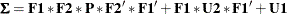where
is the 93 first-order loading matrix for the observed variables,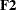is the 31 second-order loading matrix for the first-order factors,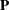is the 11 covariance matrix for the second-order factor f4,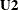is the 33 error covariance matrix of the first-order factors f1f3 (or the covariance matrix of the error terms e1012), and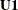is the 99 error covariance matrix for the observed variables (or the covariance matrix of the error terms e19).

Matrixcontains the loading parameters x1x9 and matrixcontains the loading parameters x10x12. Because there is only one second-order factor f4 in the model, matrixis a scalar, which is a fixed constant 1 in the LINEQS model. Matrixis an identity matrix because all error variances are fixed at 1 and they are not correlated. Matrixis a diagonal matrix that contains the parameters u1u9. Given this information, you can use the following statements to specify the second-order factor model as a COSAN model:

proc calis data=Thurst nobs=213 corr nose;
cosan
var = obs1-obs9,
F1(3) * F2(1) * P(1,IDE) + F1(3) * U2(3,IDE) + U1(9,DIA);
matrix F1
[1 , @1] = x1-x3,
[4 , @2] = x4-X6,
[7 , @3] = x7-x9;
matrix F2
[ ,1]    = x10-x12;
matrix U1
[1,1]    = u1-u9;
bounds
0. <= u1-u9;
vnames
F1 = [f1 f2 f3],
F2 = [f4],
U1 = [e1-e9];
run;


In the PROC CALIS statement, you specify the observed variables in the VAR= option and the covariance structures for the observed variables. In the terms of the covariance structure formula, you need to specify the expressions only up the central symmetric matrices. The latter parts of these expressions are redundant and can be generated automatically by PROC CALIS, as shown in Output 29.30.2.

Output 29.30.2: The Covariance Structures and Model Matrices of the Second-Order Factor Model: COSAN Model

COSAN Model Structures
Sigma = F1*F2*P*F2*F1 + F1*U2*F1 + U1

Summary of Model Matrices
Matrix N Row N Col Matrix Type
F1 9 3 GEN: Rectangular
F2 3 1 GEN: Vector
P 1 1 IDE: Identity
U1 9 9 DIA: Diagonal
U2 3 3 IDE: Identity

Output 29.30.2 shows that the intended covariance structures for the observed variables are being analyzed. The matrix types are shown next. Matrixis a rectangular matrix and matrixis a vector, although they have the default general (GEN) matrix type. Matricesandare fixed identity (IDE) matrices in the model. For these two matrices, you do not need to specify any of their elements by using the MATRIX statement because they are already well-defined with the IDE type. Lastly, matrixis a diagonal (DIA) matrix in the model.

Output 29.30.3 shows the estimates of the first-order factor loading matrix.

Output 29.30.3: Estimation of theMatrix of the Second-Order Factor Model: COSAN Model

Model Matrix F1
(9 x 3 General Rectangular Matrix)
f1 f2 f3
Obs1
 0.5151 [x1]
 0
 0
Obs2
 0.5203 [x2]
 0
 0
Obs3
 0.4874 [x3]
 0
 0
Obs4
 0
 0.5211 [x4]
 0
Obs5
 0
 0.4971 [x5]
 0
Obs6
 0
 0.4381 [x6]
 0
Obs7
 0
 0
 0.4524 [x7]
Obs8
 0
 0
 0.4173 [x8]
Obs9
 0
 0
 0.4076 [x9]

In the MATRIX statement for, you specify the pattern of the loadings. In the first entry of the MATRIX statement, you specify the loadings in the following elements: [1,1], [2,1], and [3,1]. They are free parameters x1x3, respectively. Notice that the @ sign is necessary in the first entry because the elements being defined would have been [1,1], [2,2], and [3,3] otherwise. The @ sign fixes the column number to 1. See the MATRIX statement for more details about the notation. Similarly, you define the other clusters of loading in the second and third entries in the MATRIX statement for. This explains the pattern of factor loadings in Output 29.30.3. These loading estimates x1x9 match those by the LINEQS model specification, as shown in Output 29.30.1.

Output 29.30.3 shows the estimates of the second-order factor loading matrix.

Output 29.30.4: Estimation of theMatrix of the Second-Order Factor Model: COSAN Model

Model Matrix F2
(3 x 1 Column Vector)
f4
f1
 1.4438 [x10]
f2
 1.2538 [x11]
f3
 1.4066 [x12]

In the MATRIX statement for, you do not specify the row numbers in the [ ,1] specification. PROC CALIS interprets this as stating that all the valid elements in the first column are being specified in the parameter list. In the current example, this means that elements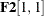,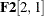, and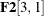are filled with the free parameters x10, x11, and x12, respectively. Output 29.30.3 shows these specification and the corresponding estimates, which match those of the LINEQS model specification, as shown in Output 29.30.1.

Output 29.30.5 shows the estimates of the error covariance matrix.

Output 29.30.5: Estimation of theMatrix of the Second-Order Factor Model: COSAN Model

Model Matrix U1
(9 x 9 Diagonal Matrix)
e1 e2 e3 e4 e5 e6 e7 e8 e9
e1
 0.1815 [u1]
 0
 0
 0
 0
 0
 0
 0
 0
e2
 0
 0.1649 [u2]
 0
 0
 0
 0
 0
 0
 0
e3
 0
 0
 0.2671 [u3]
 0
 0
 0
 0
 0
 0
e4
 0
 0
 0
 0.3015 [u4]
 0
 0
 0
 0
 0
e5
 0
 0
 0
 0
 0.3645 [u5]
 0
 0
 0
 0
e6
 0
 0
 0
 0
 0
 0.5064 [u6]
 0
 0
 0
e7
 0
 0
 0
 0
 0
 0
 0.3903 [u7]
 0
 0
e8
 0
 0
 0
 0
 0
 0
 0
 0.4814 [u8]
 0
e9
 0
 0
 0
 0
 0
 0
 0
 0
 0.5051 [u9]

In the MATRIX statement for, you specify the diagonal elements of the matrix by using the starting element at [1,1]. The parameter assignment proceeds to [2,2], [3,3] and so on such that all the trailing parameters u1u9 are filled. This means that the last element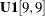is a free parameter named u9`. Output 29.30.5 confirms this intended pattern. Again, all these error variance estimates match those by the LINEQS model specification, as shown in Output 29.30.1.